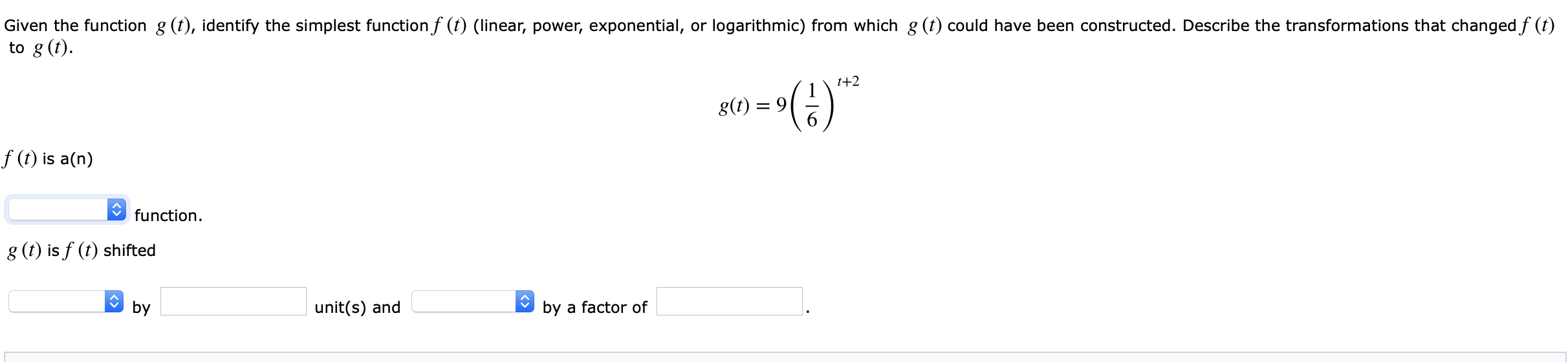# Given the function g (), identify the simplest function f (1) (linear, power, exponential, or logarithmic) from which g (t) could have been constructed. Describe the transformations that changed f (1)to g(D)4)f (0) is a(n)function.g (t) is f (t) shiftedbyunit(s) andby a factor of

Questionhelp_outlineImage TranscriptioncloseGiven the function g (), identify the simplest function f (1) (linear, power, exponential, or logarithmic) from which g (t) could have been constructed. Describe the transformations that changed f (1) to g(D) 4) f (0) is a(n) function. g (t) is f (t) shifted by unit(s) and by a factor of fullscreen
check_circleExpert Solution
Step 1

Given information:

The given function is

Step 2

Concept Used:

Stretched of the parent function f(x)=b^x

The function g(x)=ab^x is stretched vertically by a factor of a if |a|>1.

Horizontal transformation of the parent function f(x)=b^x

The function g(x)=b^(x+c) is shifted horizontally c units to the left.

Calculation:

Here, the parent function is f(t)=a^t.

The value of a is 1/6.

So, f(t)=(1/6)^t

Now, shift the parent function horizontally 2 units to the left.

Step 3

Now, stretched the above function vertica...

### Want to see the full answer?

See Solution

#### Want to see this answer and more?

Solutions are written by subject experts who are available 24/7. Questions are typically answered within 1 hour*

See Solution
*Response times may vary by subject and question
Tagged in

### Algebra